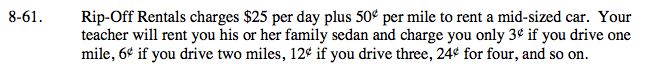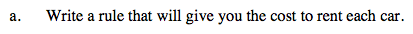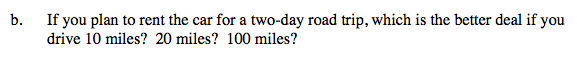### Home > A2C > Chapter 8 > Lesson 8.1.4 > Problem8-61

8-61.Let d = number of days, m = number of miles, and y = total cost.

Your teacher is using a geometric sequence.

y = 25d + 0.5m and
y = 0.03(2)m−1Substitute the values into each equation to see which rental costs less for each distance.